Next: Scalar multiplication Up: Motion in 3 dimensions Previous: Vector addition

## Vector magnitude

If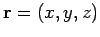represents the vector displacement of pointfrom the origin, what is the distance between these two points? In other words, what is the length, or magnitude,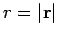, of vector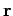. It follows from a 3-dimensional generalization of Pythagoras' theorem that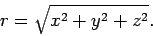(35)

Note that if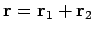then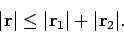(36)

In other words, the magnitudes of vectors cannot, in general, be added algebraically. The only exception to this rule (represented by the equality sign in the above expression) occurs when the vectors in question all point in the same direction. According to inequality (36), if we move 1m to the North (say) and next move 1m to the West (say) then, although we have moved a total distance of 2m, our net distance from the starting point is less than 2m--of course, this is just common sense.Next: Scalar multiplication Up: Motion in 3 dimensions Previous: Vector addition
Richard Fitzpatrick 2006-02-02